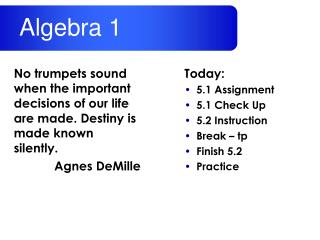DownloadDownload PresentationToday: 5.1 Assignment 5.1 Check Up 5.2 Instruction Break – tp Finish 5.2 Practice

# Today: 5.1 Assignment 5.1 Check Up 5.2 Instruction Break – tp Finish 5.2 Practice

Download Presentation## Today: 5.1 Assignment 5.1 Check Up 5.2 Instruction Break – tp Finish 5.2 Practice

- - - - - - - - - - - - - - - - - - - - - - - - - - - E N D - - - - - - - - - - - - - - - - - - - - - - - - - - -
##### Presentation Transcript

1. No trumpets sound when the important decisions of our life are made. Destiny is made known silently. Agnes DeMille Algebra 1 Today: • 5.1 Assignment • 5.1 Check Up • 5.2 Instruction • Break – tp • Finish 5.2 • Practice

2. No trumpets sound when the important decisions of our life are made. Destiny is made known silently. Agnes DeMille Algebra 1 Assignment: • 5.1 p276 #3-32 • MidTerm Progress Report Signature • Goal Evaluation

3. 5.1 CHECK UP Write the equation of the lines in slope-intercept form. 4. Your uncle weigh 200 lbs. If he loses 2 pound per month, write an equation to model this.

4. Objectives: Write linear equations given m and a point. Use linear equations in real life. Vocabulary: none Writing Linear Equations Giventhe Slope and a Point 5.2

5. Writing Linear Equations in Slope-Intercept Form 5.1 y = mx + b • How to find the Equation of a Line: • Find the slope (m) of the line. • Find the y-intercept (b) of the line. • Plug these values into y = mx + b

6. Writing Linear Equations in Slope-Intercept Form 5.2 y = mx + b • How to find the Equation of a Line: • Find the slope (m) of the line. • Find the y-intercept (b) of the line. • Plug m and a point into the equation • Plug these values into y = mx + b

7. Example 1 Writing an Equation of a Line Write the equation of the line whose slope m = -2 and passes through the point (6, -3). Find the equations of the lines: y = -2x + 9

8. 7 6 5 4 3 2 1 -6 -4 -2 1 2 3 4 5 6 7 -2 -4 -6 Example 2 Writing an Equation of a Line from a Graph Write the equation of the line shown in the graph.

9. Example 3 Writing an Equation of Parallel Lines Write the equation of the line that is parallel to the line y = -3x – 2 and passes through the point (3, -4) Find the equations of the lines: y = -3x + 5

10. Example 4 Real Life Linear Models Between 1995 and 2005, the number of vacation trips in the U.S. take by U.S. residents increased by about 26 million per year. In 2003, U.S. residents went on 740 million vacation trips. Write linear equation the models the number of vacation trips, y (in millions), in terms of the year, t. Let t be the number of years from 1995. Estimate the number of trips in 2015. y = 26t + 532 ≈ 1052 million trips

11. Example 4 Real Life Linear Models All the employees of a garden center are given a \$.40 per hour raise each year. You make \$7.15 per hour after three years as an employee. Write a linear equation that models your salary per hour S in terms of n the number of years you have worked at the garden center. Then find your hourly salary after 6 years. S = .4n + 5.95 \$8.35/hr

12. Practice 5.2 Assignment 5.1 p282 #3-11,…

13. No trumpets sound when the important decisions of our life are made. Destiny is made known silently. Agnes DeMille Algebra 1 Assignment: • 5.2 p282 #5-11, 13-35 odd, 42-45 • MidTerm Progress Report Signature • Goal Evaluation

14. Supply Check • textbook • pencil • binder w/dividers • paper • graph paper • calculator • colored pencils